### Standing Waves in a Chain Vertically Hung

 Standing Waves in a Chain Vertically HungIn the lowest order, the velocity of transverse waves in a chain freely hanging maybe given by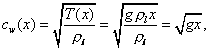where x is the distance from the lower, free end of the chain. The wave velocity is stronglydependent on the location and the chain can be regarded as a typical nonuniform wave medium. The differential equation to describe transverse waves in the chain is given by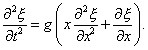(See Note No. 8 for detailed derivation.) This is not in the form of standard wave equation. For a narrow pulse, the term of first order derivative can be ignored,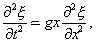which yields the approximated propagation velocity given in the first equation.  Standing wave in the form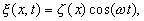satisfies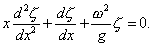This can be satisfied by the zero-th order Bessel function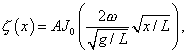where L is the length of he chain. At the upper end x = L, the displacement should vanish. The Bessel function J0(y) becomes zero when y = .4048, 5.5201, 8.6537, ?. Therefore, the resonance frequencies are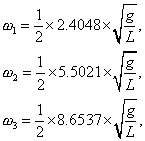and so on. Animation shows standing wave patterns with those frequencies.Standing waves in a chain vertically hung (typical nonuniform wave medium). Lowest order mode. > with(plots): animate([.1*BesselJ(0,2.4048*sqrt(x))*sin(t),x,x=0..1],t=0..2*Pi,frames=60,color=red);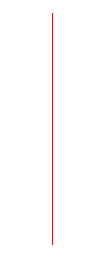Second mode. > animate([BesselJ(0,5.5201*sqrt(x))*sin(1.81*t),x,x=0..1],t=0..2*Pi/1.81,frames=30, color=red);Third mode. > animate([BesselJ(0,8.6537*sqrt(x))*sin(3.6*t),x,x=0..1],t=0..2*Pi/3.6,frames=20, color=red);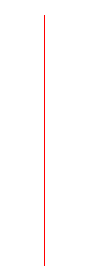Ċ
Jason Lin,
2018年9月6日 凌晨12:42